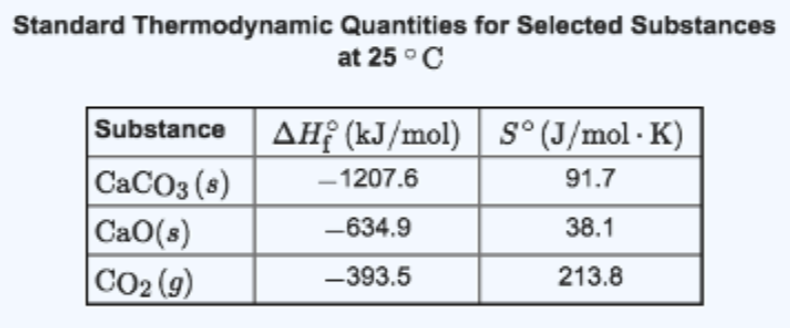# Problem: Consider the reaction. CaCO3 (s) → CaO (s) + CO2 (g) Estimate ΔG° (kJ) for this reaction at each temperature. (Assume that ΔH° and ΔS° do not change too much within the given temperature range.) Part A:  290 K Express your answer using one decimal place. Part B :  1065 K Express your answer using one decimal place. Part C:  1445 K Express your answer using one decimal place.

###### FREE Expert Solution
91% (223 ratings)
###### FREE Expert Solution
91% (223 ratings)###### Problem Details

Consider the reaction.

CaCO3 (s) → CaO (s) + CO2 (g)

Estimate ΔG° (kJ) for this reaction at each temperature. (Assume that ΔH° and ΔS° do not change too much within the given temperature range.)Part A:  290 K

Express your answer using one decimal place.

Part B :  1065 K

Express your answer using one decimal place.

Part C:  1445 K

Express your answer using one decimal place.

Frequently Asked Questions

What scientific concept do you need to know in order to solve this problem?

Our tutors have indicated that to solve this problem you will need to apply the Gibbs Free Energy concept. You can view video lessons to learn Gibbs Free Energy. Or if you need more Gibbs Free Energy practice, you can also practice Gibbs Free Energy practice problems.

What is the difficulty of this problem?

Our tutors rated the difficulty ofConsider the reaction. CaCO3 (s) → CaO (s) + CO2 (g) Estimat...as high difficulty.

How long does this problem take to solve?

Our expert Chemistry tutor, Dasha took 12 minutes and 6 seconds to solve this problem. You can follow their steps in the video explanation above.

What professor is this problem relevant for?

Based on our data, we think this problem is relevant for Professor Enderle's class at UCD.